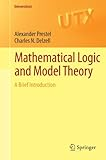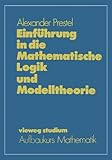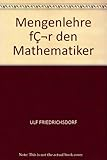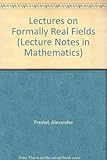search for books and compare prices
Alexander Prestel has written 6 work(s)
Search for other authors with the same name
displaying 1 to 6 | at end
show results in order: alphabetically | oldest to newest | newest to oldestProduct Description: Mathematical Logic and Model Theory: A Brief Introduction offers a streamlined yet easy-to-read introduction to mathematical logic and basic model theory. It presents, in a self-contained manner, the essential aspects of model theory needed to understand model theoretic algebra...read more

Paperback:

9781447121756 | Springer Verlag, September 7, 2011, cover price \$59.95 | About this edition: Mathematical Logic and Model Theory: A Brief Introduction offers a streamlined yet easy-to-read introduction to mathematical logic and basic model theory.

Product Description: Absolute values and their completions â such as the p-adic number fields â play an important role in number theory. Krull's generalization of absolute values to valuations made possible applications in other branches of mathematics...read more

Paperback:

9783642063459 | Springer Verlag, December 15, 2010, cover price \$109.00 | About this edition: Absolute values and their completions â such as the p-adic number fields â play an important role in number theory.

Product Description: Positivity is one of the most basic mathematical concepts, involved in many areas of mathematics (analysis, real algebraic geometry, functional analysis, etc.). The main objective of the book is to give useful characterizations of polynomials...read more

Hardcover:

9783540412151 | Springer Verlag, June 1, 2001, cover price \$99.00 | About this edition: Positivity is one of the most basic mathematical concepts, involved in many areas of mathematics (analysis, real algebraic geometry, functional analysis, etc.

Paperback:

9783642074455, titled "Positive Polynomials: From Hilbertâs 17th Problem to Real Algebra" | Springer Verlag, September 22, 2011, cover price \$99.00 | About this edition: Positivity is one of the most basic mathematical concepts, involved in many areas of mathematics (analysis, real algebraic geometry, functional analysis, etc.

Product Description: Ein wesentliches Ziel dieses Buches ist, Studenten des Hauptstudiums und interessierten Mathematikern die MÃ¶glichkeit zu erÃ¶ffnen, die bekanntesten, in der Algebra zur Zeit Ã¼blichen modelltheoretischen SchlÃ¼sse kennen und verstehen zu lernen...read more

Paperback:

9783528072605, titled "EinfÃ¼hrung in Die Mathematische Logik Und Modelltheorie" | Springer Verlag, January 1, 1986, cover price \$69.99 | About this edition: Ein wesentliches Ziel dieses Buches ist, Studenten des Hauptstudiums und interessierten Mathematikern die MÃ¶glichkeit zu erÃ¶ffnen, die bekanntesten, in der Algebra zur Zeit Ã¼blichen modelltheoretischen SchlÃ¼sse kennen und verstehen zu lernen.

Product Description: Das vorliegende Blichlein ist aus Vorlesungen hervorgegangen, die wir abwechselnd an der Universitat Konstanz hielten und noch immer halten. Die Absicht dieser Vor lesung ist es, Mathematikstudenten mittlerer Semester einen Einblick in die Mengen lehre zu vermitteln, der ihnen gleichzeitig die flir die Mathematik wichtigsten mengen theoretischen Begriffe und Satze an die Hand gibt...read more

Paperback:

9783528072582 | Springer Verlag, April 5, 2012, cover price \$69.95 | About this edition: Das vorliegende Blichlein ist aus Vorlesungen hervorgegangen, die wir abwechselnd an der Universitat Konstanz hielten und noch immer halten.

displaying 1 to 6 | at end